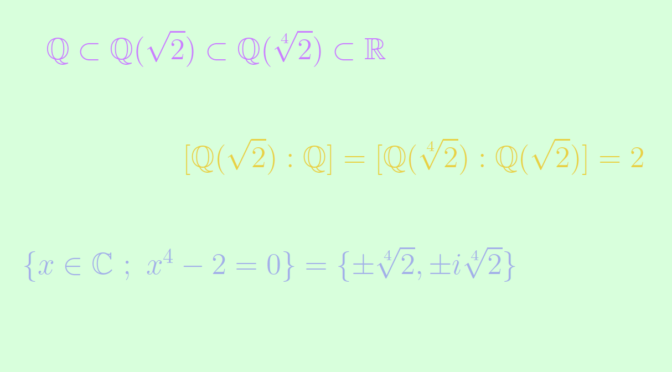# A normal extension of a normal extension may not be normal

An algebraic field extension $$K \subset L$$ is said to be normal if every irreducible polynomial, either has no root in $$L$$ or splits into linear factors in $$L$$.

One can prove that if $$L$$ is a normal extension of $$K$$ and if $$E$$ is an intermediate extension (i.e., $$K \subset E \subset L$$), then $$L$$ is a normal extension of $$E$$.

However a normal extension of a normal extension may not be normal and the extensions $$\mathbb Q \subset \mathbb Q(\sqrt{2}) \subset \mathbb Q(\sqrt{2})$$ provide a counterexample. Let’s prove it.

As a short lemma, we prove that a quadratic extension $$k \subset K$$ , i.e. an extension of degree two is normal. Suppose that $$P$$ is an irreducible polynomial of $$k[x]$$ with a root $$a \in K$$. If $$a \in k$$ then the degree of $$P$$ is equal to $$1$$ and we’re done. Otherwise $$(1, a)$$ is a basis of $$K$$ over $$k$$ and there exist $$\lambda, \mu \in k$$ such that $$a^2 = \lambda a +\mu$$. As $$a \notin k$$, $$Q(x)= x^2 – \lambda x -\mu$$ is the minimal polynomial of $$a$$ over $$k$$. As $$P$$ is supposed to be irreducible, we get $$Q = P$$. And we can conclude as $Q(x) = (x-a)(x- \lambda +a).$

The entensions $$\mathbb Q \subset \mathbb Q(\sqrt{2})$$ and $$\mathbb Q(\sqrt{2}) \subset \mathbb Q(\sqrt{2})$$ are quadratic, hence normal according to previous lemma and $$\sqrt{2}$$ is a root of the polynomial $$P(x)= x^4-2$$ of $$\mathbb Q[x]$$. According to Eisenstein’s criterion $$P$$ is irreducible over $$\mathbb Q$$. However $$\mathbb Q(\sqrt{2}) \subset \mathbb R$$ while the roots of $$P$$ are $$\pm \sqrt{2}, \pm i \sqrt{2}$$ and therefore not all real. We can conclude that $$\mathbb Q \subset \mathbb Q(\sqrt{2})$$ is not normal.